Published: 10 October 2014

# Dynamics of vibroprotecting system when using controlled damping fluids with hereditary factor

G. N. Reizina1
A. Bubulis2
E. V. Korobko3
G. K. Voronovich4
1, 3, 4A.V. Luikov Heat and Mass Transfer Institute of NAS Belarus, Minsk, Belarus
2Kaunas University of Technology, Kaunas, Lithuania
Corresponding Author:
E. V. Korobko
Views 18

#### Abstract

Oscillating system with a damper containing controlled damping fluid with hereditary electrorheological characteristics was investigated. Numerical calculation of the system in quasistationary approximation was carried out to prove the accuracy of applied assumptions.

## 1. Introduction

One of the promising methods for targeted reduction of vibration exposure on the object is its vibroisolation, in particular, with the use of viscous damping. This method finds new control features through the use of modern intellectual damping materials with electric and magnetosensitive nanofillers [1, 2]. In the electrorheological materials (ERF) containing non-colloidal particles as the dispersed phase, under the influence of external field a strong structuring of particles is observed. Thanks to it, the material exhibits elastic and viscoplastic properties according to the level of external influence. This allows one to control its damping characteristics specifically and reversibly (response time less than 10-3 s). It is known, that ERFs are used as fluids for flow hydraulic systems and fluid dampers, squeeze dampers [3-5], including ground transportation systems and precision instruments. Analysis of rheological behavior of non-Newtonian ERFs shows that the hereditary factor becomes important , when the duration of the relaxation processes occurring in an environment commensurates with the time scale of hydrodynamic phenomena. For the considered electrosensitive flowing dispersions relaxation times are in the range from 10-2 to 10 s and heredity is manifested in conventional hydrodynamic regimes such as characteristic for the flow of the damping fluid in the channel of an electric shock absorber in the suspension system of the vehicle. The present paper is devoted to the modeling of oscillating system with ERF-damping in the tracking mode with the hereditary factor.

## 2. Main part

Consider the effect of the rheological features of hereditary media on the dynamics of vibroprotecting system of the damping device, shown in Fig. 1.

Fig. 1Scheme of damping device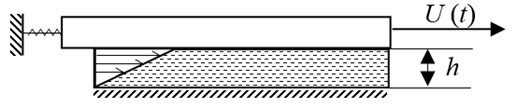For example, in the damping device, the gap width $h$ is much less than the channel width and radius of curvature of the wall, $Re\ll$1, we neglect the change in the shear stress across the gap can be neglected. Then the equation of motion of a body with mass $m$, connected to a damper, can be written as follows:

1
$m\stackrel{¨}{X}+S\tau \left(\stackrel{˙}{\gamma }\right)+\xi X=0,\mathrm{}\mathrm{}\stackrel{˙}{\gamma }=\frac{X-U\left(t\right)}{h},\mathrm{}\mathrm{}\mathrm{}X\left(0\right)=\stackrel{˙}{X}\left(0\right)=0$

where $X$ – the displacement of the body connected to the spring; $S$ – the surface wetted by the fluid; $\stackrel{˙}{\gamma }$ – the shear rate; $\tau$ – the shear stress; $\xi$ – the spring rigidity.

Dependence of the shear stress versus the shear rate for nonlinear elastic electrorheological fluid is defined as:

2
$\tau =\sum _{i=1}^{\mathrm{\infty }}{\tau }_{i}\left(t\right),\frac{d{\tau }_{k}}{dt}+\frac{{q}_{k}}{{\lambda }_{k}}{\tau }_{k}={P}_{k}\stackrel{˙}{\gamma },\frac{d{P}_{k}}{dt}+\frac{{q}_{k}}{{\lambda }_{k}}{P}_{k}=\frac{{\eta }_{k}}{{{\lambda }_{k}}^{2}}{f}_{k},$

where functions ${q}_{k}$ and ${f}_{k}$ depend on the instantaneous value of the shear rate $\stackrel{˙}{\gamma }\left(t\right)$; ${\eta }_{k}$, ${\lambda }_{k}$ – characteristics of the spectrum of relaxation times in the linear viscoelastic region. Values ${q}_{k}\left(\stackrel{˙}{\gamma }\right)$ and ${f}_{k}\left(\stackrel{˙}{\gamma }\right)$ incorporate the change of the shear modulus and relaxation time of the fluid with the increase of shear rate.

Transition to dimensionless quantities in Eqs. (1), (2) yields:

$X=\frac{{U}_{0}}{{\omega }_{0}}\stackrel{-}{X},\mathrm{}\mathrm{}\mathrm{}t={\omega }_{0}\stackrel{-}{t},\mathrm{}\mathrm{}\mathrm{}\stackrel{˙}{\gamma }=\frac{{U}_{0}}{h}\stackrel{˙}{\stackrel{-}{\gamma }},\mathrm{}\mathrm{}\mathrm{}\tau =\mu \frac{{U}_{0}}{h}\stackrel{-}{\tau },\mathrm{}\mathrm{}\mathrm{}{P}_{k}=\frac{\eta }{\lambda }{\stackrel{-}{P}}_{k},$

where ${\omega }_{0}=\sqrt{x/m}$ – the natural frequency of the body; ${U}_{0}$ – velocity scale. The dynamics of the oscillating system and established characteristics of fluid flow are defined by the following dimensionless complex: $\epsilon ={S}_{\eta }/m{\omega }_{0}h$ – damping factor.

It characterizes the ratio of viscous and inertial forces in the oscillating system and depends on :

• Modified Deborah number, which takes into account the relaxation processes in the fluid $De=\lambda {\omega }_{0}$, which is the ratio of the maximum relaxation time of the fluid to the period of natural oscillations of the system;

• Weissenberg number, showing the effect of shear rate on the rheological properties of the fluid $We=\lambda \cdot \stackrel{˙}{\gamma }$;

• As well as rheological parameters of damping fluid $\alpha$ and $\alpha \text{'}/\lambda$.

The problem task in this case is as follows:

3
$X=-E\left[\sum _{K=1}^{{K}^{\mathrm{*}}}{\stackrel{-}{T}}_{k}+\left(\sum _{K={K}^{\mathrm{*}}+1}^{NN}\frac{1}{\xi \left(\alpha \right)}\cdot \frac{1}{{K}^{\alpha }}\cdot \frac{f\left(We,{\lambda }_{k},\mathrm{}\stackrel{˙}{\gamma }\right)}{{q}^{2}\mathrm{}We,{\stackrel{-}{\lambda }}_{k},\mathrm{}\stackrel{˙}{\stackrel{-}{\gamma }}}\right)\mathrm{}\mathrm{}\mathrm{}\stackrel{˙}{\stackrel{-}{\gamma }}\right]-\stackrel{-}{X},$
${\stackrel{˙}{\stackrel{-}{T}}}_{k}=-\frac{1}{D{e}_{0}}\frac{q\left(We,\mathrm{}\overline{{\lambda }_{k}},\mathrm{}\stackrel{˙}{\stackrel{-}{\gamma }}\right)}{{\lambda }_{k}}\cdot {\stackrel{-}{T}}_{k}+\frac{1}{D{e}_{0}}{\stackrel{-}{P}}_{k}\mathrm{}\stackrel{˙}{\stackrel{-}{\gamma }},$
${\stackrel{˙}{\stackrel{-}{P}}}_{k}=-\frac{1}{D{e}_{0}}\frac{q\left(We,\mathrm{}\overline{{\lambda }_{k}},\mathrm{}\stackrel{˙}{\stackrel{-}{\gamma }}\right)}{{\lambda }_{k}}\cdot {\stackrel{-}{P}}_{k}+\frac{1}{D{e}_{0}\xi \left(\alpha \right)}\mathrm{}\frac{{\stackrel{-}{\eta }}_{k}}{{{\stackrel{-}{\lambda }}_{k}}^{2}}\mathrm{}f\left(We,\mathrm{}\overline{{\lambda }_{k}},\mathrm{}\stackrel{˙}{\stackrel{-}{\gamma }}\right),$
$\stackrel{˙}{\stackrel{-}{\gamma }}=\stackrel{˙}{\stackrel{-}{X}}-\stackrel{-}{U}\left(\stackrel{-}{t}\right),\mathrm{}\mathrm{}\mathrm{}\mathrm{}{K}^{\mathrm{*}}=6,\mathrm{}\mathrm{}\mathrm{}\stackrel{-}{t}=0,\mathrm{}\mathrm{}\mathrm{}\mathrm{}\stackrel{-}{X}=\stackrel{˙}{\stackrel{-}{X}}=0,\mathrm{}\mathrm{}\mathrm{}{\stackrel{-}{T}}_{k}=0,\mathrm{}\mathrm{}\mathrm{}\mathrm{}{\stackrel{-}{P}}_{k}=\frac{1}{\xi \left(\alpha \right)},$
${\stackrel{-}{\lambda }}_{k}={\stackrel{-}{\eta }}_{k}=\frac{1}{{K}^{\alpha }},\mathrm{}\mathrm{}\mathrm{}\mathrm{}\xi \left(\alpha \right)=\sum _{\upsilon =1}^{\mathrm{\infty }}\mathrm{}\mathrm{}\frac{1}{{\upsilon }^{\alpha }}.$

Calculations are carried out for rheological equation of state (RES) of Macdonald-Berd-Carro:

4
${f}_{k}=\frac{1-{\lambda }_{k}^{\text{'}}\left|\stackrel{˙}{\gamma }\right|}{1+{\lambda }_{k}\left|\stackrel{˙}{\gamma }\right|},\mathrm{}\mathrm{}\mathrm{}\mathrm{}{q}_{k}=\frac{{\left(1-{\lambda }_{k}^{\text{'}}\left|\stackrel{˙}{\gamma }\right|\right)}^{3/2}}{{\left(1+{\lambda }_{k}\left|\stackrel{˙}{\gamma }\right|\right)}^{1/2}},$
${\lambda }_{k}=\frac{\lambda }{{k}^{\alpha }},\mathrm{}\mathrm{}\mathrm{}\mathrm{}{\lambda }_{k}^{\text{'}}=\frac{\lambda \mathrm{\text{'}}}{{K}^{\alpha }},{\eta }_{k}=\frac{\eta }{E\left(\alpha \right){K}^{\alpha }},\mathrm{}\mathrm{}\mathrm{}\mathrm{}\frac{\lambda \mathrm{\text{'}}}{\lambda }=0,\mathrm{}2,$

where $\alpha$ – the parameter of distribution of relaxation time spectrum in the linear area; $\eta$ − initial Newtonian viscosity; $\xi \left(\alpha \right)$ – Riemann zeta-function. The ratio $\lambda \mathrm{\text{'}}/\lambda$ characterizes the influence of shear rate on shear modulus. When $\lambda \mathrm{\text{'}}=\lambda$, ${f}_{k}=\text{1}$ the stationary shear viscosity in the model Eq. (4) is defined by the dependence:

5
$\eta \left(\stackrel{˙}{\gamma }\right)=\frac{\eta }{\xi \left(\mathrm{\alpha }\right)}\sum _{K=1}^{\mathrm{\infty }}\frac{{K}^{\alpha }}{\left({K}^{\alpha }+\lambda \mathrm{\text{'}}\left|\stackrel{˙}{\gamma }\right|{\right)}^{2}}.$

In the numerical calculation of the row of Eq. (3), the first ${K}^{*}$ members are determined from differential equations, and the rest are considered already relaxed. This simplification applies when the time scale of hydrodynamic phenomena is substantially bigger then $\lambda /\left({K}^{\mathrm{*}}+1{\right)}^{\alpha }$.

When $D{e}_{0}\ll$1, the elasticity of the fluid does not appear, the parameter $\alpha$ determines only dependence of viscosity on the shear rate, i.e. the degree of “non-linearity” of the oscillating system. In this case, it has the same effect as the parameter $p$ in simplified nonlinear viscous model.

When $D{e}_{0}\gg$1, the value of $\alpha$ determines the fraction of the reversible component of the total amount of deformation of the fluid.

We carry out calculation of the dynamics of the oscillating system with ERF, rheological parameters of which depend on the strength of the external electric field $E$ with correction factor $K\left(E\right)$:

6
$\eta \left(E\right)=\frac{\eta \left(E\right)}{{\left[1+{\lambda }_{0}^{2}\left(E\right){\stackrel{˙}{\gamma }}^{2}\right]}^{p}}=\frac{\eta \cdot {K}_{1}\left(E\right)}{{\left[1+\left({\lambda }_{0}{K}_{2}\left(E\right){\right)}^{2}\right]}^{p}},\eta =\eta \cdot {K}_{1}\left(E\right),\mathrm{}\mathrm{}\mathrm{}\mathrm{}\lambda ={\lambda }_{0}\cdot {K}_{2}\left(E\right).$

Previous experimental studies  showed that the greatest effect of damping is achieved when the viscous component of ERF has the largest value. For a given fluid, this is achieved at $E=$0.3.

Increased viscosity results in an increase of the damping characteristics of the oscillating system. The growth of the electric field intensity also leads to an increase in the elastic component of ERF (Fig. 2), and hence to a decrease in the characteristic relaxation time $\lambda$.

Fig. 2Dependence of rheological parameters of elastic modulus G’ and loss modulus G’’ on the electric field intensity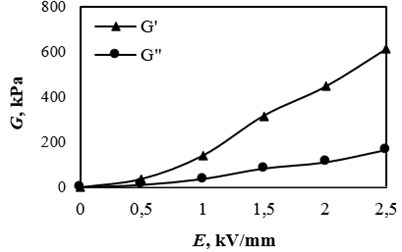Assuming proportional dependence on the growth of the effective viscosity $\eta$ and decreasing of the characteristic relaxation time $\lambda$, we obtain a quantitative relationship (see Fig. 3).

Voltage is applied in the tracking mode of the dynamic characteristics of the system, previously proposed in .

Test calculations were performed (Fig. 4) for oscillating system with the external action depending on the electric field varying in the form of a rectangular pulse.

Accounting for the effects of rheological properties of the fluid dynamics of shear flow was carried out in a wide range of rheological parameters: $\epsilon =$0.314-3.14; $\lambda \text{'}/\lambda =$ 0.2; $\alpha =$2-3; ${W}_{e}=$ 0-100; $D{e}_{0}=$0.1-5.0 and $E=$0-0.3 kV/mm. Calculations were carried out at ${K}^{\mathrm{*}}=\mathrm{}$6 to increase the count rate, since the difference in the numerical values obtained for ${K}^{\mathrm{*}}=\mathrm{}$23 and ${K}^{\mathrm{*}}=\mathrm{}$6 is only 2 %.

Fig. 3Qualitative characteristics of elastic a(E) and viscous b(E) components of ERF, [E] – kV/mm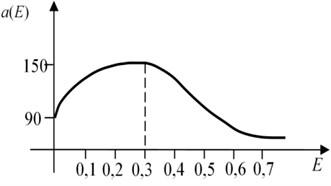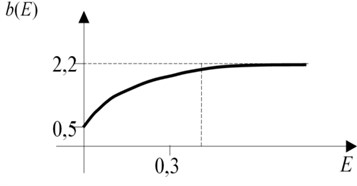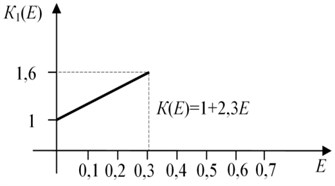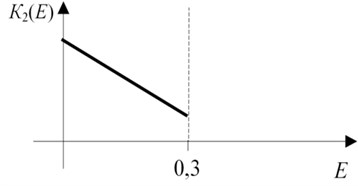Fig. 4Characteristics of established oscillations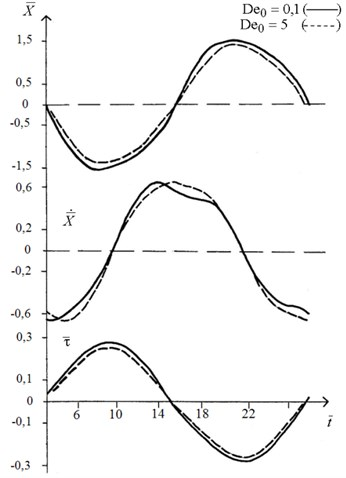Thus the rheological model of MacDonald-Berd-Carro used in the calculation predicts the nonlinear behavior of the system. Indeed, the calculation results for the nonlinear viscous rheological equation of MacDonald-Berd-Carro are in good agreement with the results of calculations for a simple nonlinear viscous model:

$\eta \left(\stackrel{˙}{\gamma }\right)=\frac{\eta }{\left(1+{\lambda }^{2}{\stackrel{˙}{\gamma }}^{2}{\right)}^{p}},p<0,5.$

Thus, the accuracy of the data in the quasistationary approximation is shown, which can dramatically reduce the time of calculating the characteristics of the oscillating system.

## 3. Conclusions

Studies performed have shown that the change in the controlling of the external impact by the electric field in the tracking mode leads to a change of the elastic and viscous components of the damping fluid, as well as the number of parameters of Deborah and Weissenberg numbers, and, consequently, to a change in the damping coefficient, which in turn affects the dynamics of the oscillating system.